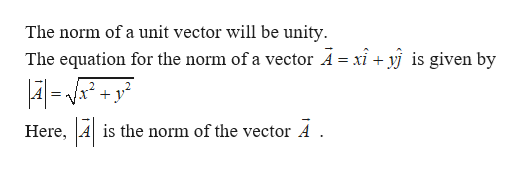If A = a(3.0i + 4.0j), where a is a constant, determine the value of a that makes A a unit vector.

Question

If Aa(3.0i + 4.0j), where a is a constant, determine the value of a that makes A a unit vector.

Step 1help_outlineImage TranscriptioncloseThe norm of a unit vector will be unity The equation for the norm of a vector A= xi+ yj is given by -y2 + = Here is the norm of the vector A . fullscreen

Want to see the full answer?

See Solution

Want to see this answer and more?

Our solutions are written by experts, many with advanced degrees, and available 24/7

See Solution
Tagged in

Vectors and Scalars